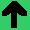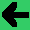# Lab 21: Parallelization

1. It's typically thought that it takes at least O(NlogN) time to sort an array.
2. However, if you do it in parallel, you can do it in N time.
3. The algorithm is that each process is connected to both its neighbors (except the end ones).
4. In alternating steps each process looks right then left (lets' say the odds start with right and the evens with left).
5. If the left is larger than the right, switch.
6. This will sort in N time. (Though there still O(NlogN) switches).
7. Implement this. You are welcome to do it how you like, but I suggest
1. Put in an unsorted array of say 10 numbers.
2. Write a print function to print the array.
3. Now, simulate processes by calling a function with the two process numbers.
4. Implement a pass function between two adjacent processes.
5. Loop through 10 times calling the processes, and printing out the array.
6. If you've got this far, make it work for 100 numbers with randomly initialised numbers.
7. If you've got this far, actually fork off processes.